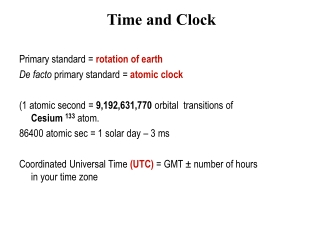DownloadDownload PresentationTime and Clock

# Time and Clock

Download Presentation## Time and Clock

- - - - - - - - - - - - - - - - - - - - - - - - - - - E N D - - - - - - - - - - - - - - - - - - - - - - - - - - -
##### Presentation Transcript

1. Primary standard = rotation of earth De facto primary standard = atomic clock (1 atomic second = 9,192,631,770 orbital transitions of Cesium 133 atom. 86400 atomic sec = 1 solar day – 3 ms Coordinated Universal Time (UTC) = GMT ± number of hours in your time zone Time and Clock

2. Global positioning system: GPS Location and precise time computed by triangulation Right now GPS time is nearly 14 seconds ahead of UTC, since It does not use leap sec. correction Per the theory of relativity, an additional correction is needed. Locally compensate by the Receivers. A system of 24 satellites (now 31) broadcast accurate spatial corordinates and time maintained by atomic clocks

3. Happening at the same time? NO. There is nothing called simultaneous in the physical world. What does “concurrent” mean? Alice Explosion 2 Explosion 1 Bob

4. Sequential and Concurrent events Sequential = Totally ordered in time. Total ordering is feasible in a single process that has only one clock. This is not true in a distributed system. Two issues are important here: • How to synchronize physical clocks ? • Can we define sequential and concurrent events without using physical clocks?

5. Causality Causality helps identify sequential and concurrent events without using physical clocks. Joke  Re: joke ( implies causally ordered before or happened before) Message sent  message received Local ordering a  b  c (based on the local clock)

6. Defining causal relationship Rule 1. If a, b are two events in a single process P, and the time of a is less than the time of b then a b. Rule 2. If a = sending a message, and b = receipt of that message, then a  b. Rule 3.a  bb  c a  c

7. a  d since (a  bb  cc  d) e  d since (e  ff  d) (Note that  defines a PARTIAL order). Is g f or f g? NO.They are concurrent. . Example of causality Concurrency = absence of causal order

8. LC is a counter. Its value respects causal ordering as follows a  b  LC(a) < LC(b) Note that LC(a) < LC(b) does NOT imply a  b. Each process maintains its logical clock as follows: LC1. Each time a local event takes place, increment LC. LC2. Append the value of LC to outgoing messages. LC3. When receiving a message, set LC to 1 + max (local LC, message LC) Logical clocks

9. Total order is important for some applications like scheduling (first-come first served). But total order does not exist! What can we do? Strengthen the causal order to define a total order (<<) among events. Use LC to define total order (in case two LC’s are equal, process id’s will be used to break the tie). Let a, b be events in processes i and j respectively. Then a << b iff -- LC(a) < LC(b) OR -- LC(a) = LC(b) and i < j a  b  a << b, but the converse is not true. Total order in a distributed system The value of LC of an event is called its timestamp.

10. Causality detection can be an important issue in applications like group communication. Logical clocks do not detect causal ordering. Vector clocksdo. a  b  VC(a) < VC(b) Vector clock C may receive Re:joke before joke, which is bad!

11. {Actions of process j} 1. Increment VC[j]) for each local event. 2. Append the local VCto every outgoing message. 3. When a message with a vector timestamp Tarrives, first increment the jth component VC[j] of the local vector clock, and then update it as follows: k: 0 ≤ k ≤N-1:: VC[k] := max (T[k], VC[k]). Implementing VC jth component of VC

12. Example [3, 3, 4, 5, 3, 2, 1, 4] < [3, 3, 4, 5, 3, 2, 2, 5] But, [3, 3, 4, 5, 3, 2, 1, 4] and [3, 3, 4, 5, 3, 2, 2, 3] are not comparable Vector clocks Let a, b be two events. Define. VC(a) < VC(b) iff i : 0 ≤ i ≤ N-1 : VC(a)[i] ≤ VC(b)[i], and  j : 0 ≤ j ≤ N-1 : VC(a)[j] < VC(b)[j], VC(a) < VC(b)  a  b Causality detection